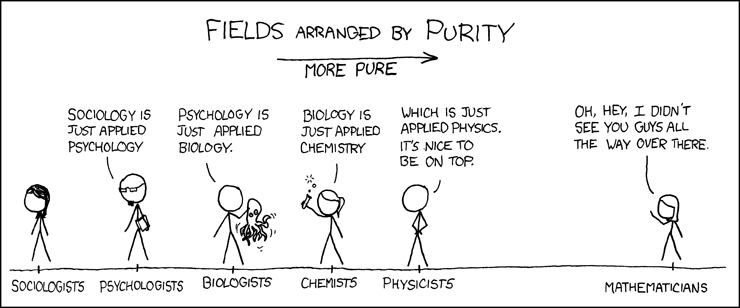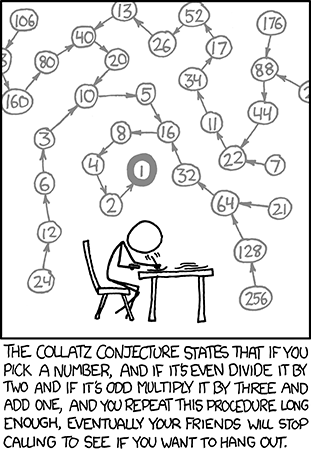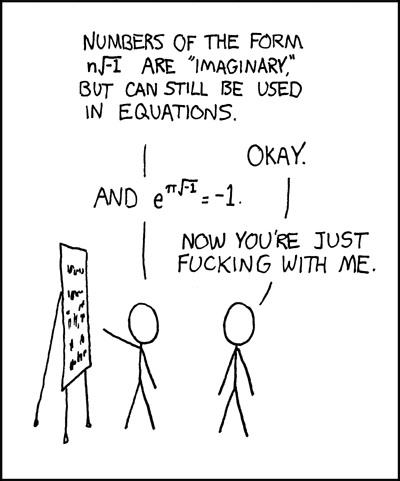You are currently browsing the tag archive for the ‘humor’ tag.1. Not only is math beautiful, it is$\int e^{xy}$.
2. Why did the chicken cross the Möbius Strip?
3. I find your lack of math disturbing.
4.$\sqrt{-1}$$2^{3}$$\sum$$\pi$…and it was delicious.
5. Mathematical puns are the first sine of madness.
6. If I’ve told you$n$ times, I’ve told you$n+1$ times…
7. I have an imaginary friend$\sqrt{-1}$.
8. A mathematician is a machine for turning coffee into theorems. – Alfréd Rényi
9. Instant mathematician just add coffee.
10. Five out of four people don’t understand fractions.
11. Old mathematicians never die. They just lose some of their functions.
12. A topologist is a person who doesn’t know the difference between a coffee cup and a doughnut.
13. Mathematicians have problems.
15. With mathematics, the possibilities are infinite.
16.$i^{2}$, I keep it real.
17. Be happy. Do math.
18. Mathematics is the language God used to write the universe.
19. A mathematician, like a painter or poet, is a maker of patterns. If his patterns are more permanent than theirs, it is because they are made with ideas. – G. H. Hardy
20. Mathematics knows no races or geographic boundaries; for mathematics, the cultural world is one country. – David Hilbert
21. What is the volume of a disk with radius$z$ and height$a$?$Pi \cdot z \cdot z \cdot a$.Riker and Picard discuss Fermat’s Last Theorem in “The Royale.” Captain Picard: ‎”For 800 years people have been trying to solve it (Fermat’s Last Theorem)…”

Andrew Wiles did solve it after more than 350 years after Fermat first mentioned the proposition in the margin of his Arithmetica in 1637. Wiles proved FLT in 1994 and it was published in 1995. Apparently, the writers of ST TNG thought this theorem was completely inaccessible.View the video here.Euler Formula

The Euler formula, sometimes also called the Euler identity, states$\displaystyle e^{ix}=cosx+isinx,$

where${i}$ is the imaginary unit. Note that Euler’s polyhedral formula is sometimes also called the Euler formula, as is the Euler curvature formula.

The special case of the formula with${x=\pi}$ gives the beautiful identity$\displaystyle e^{i\pi}=-1,$

an equation connecting the fundamental numbers${i,\pi,e,1,}$ and${0}$, the fundamental operations${+}$,${\times}$, and exponentiation, the most important relation${=}$, and nothing else. Gauss is reported to have commented that if this formula was not immediately obvious, the reader would never be a first-class mathematician.

This is an image of a pdf document that was created out of$Latex$.